# College Chemistry : Reaction Coordinate Diagrams

## Example Questions

### Example Question #11 : College Chemistry

Given the following reaction diagram, which of the following is marked by the red arrow?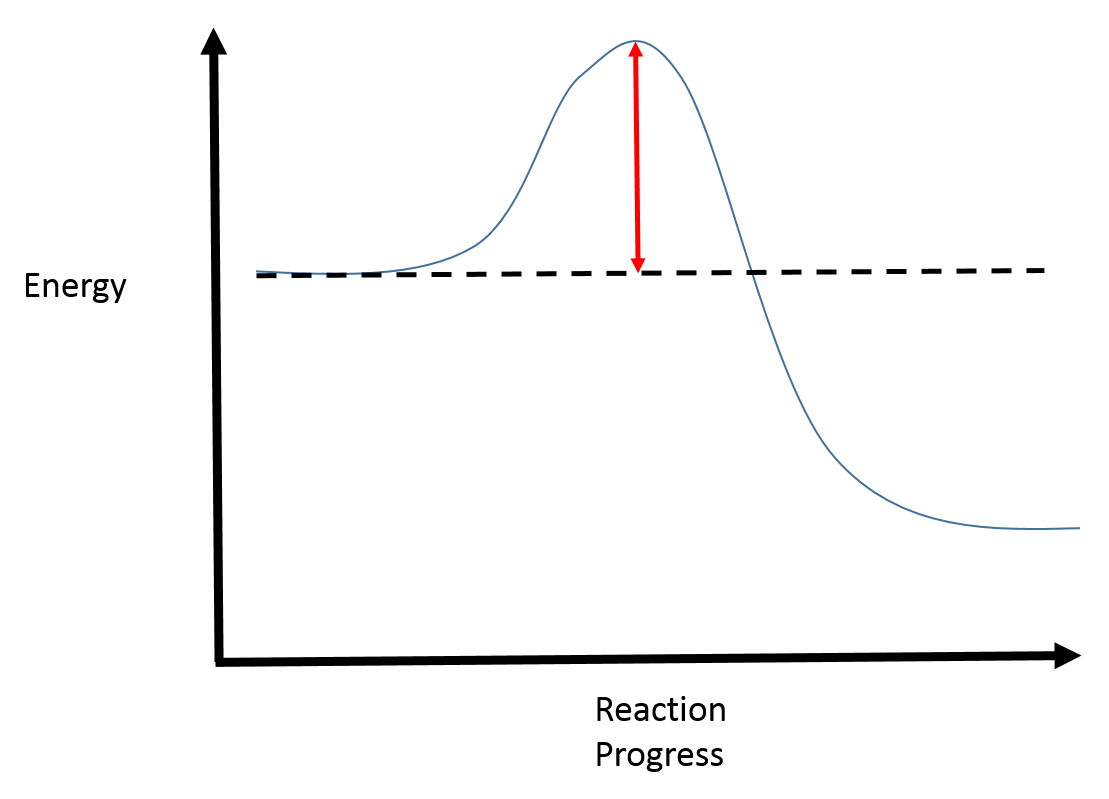Activation energy for the reverse reaction

Activation energy for the forward reaction

Change in enthalpy

Change in entropy

Activation energy for the forward reaction

Explanation:

The fully filled in reaction coordinate diagram is displayed below.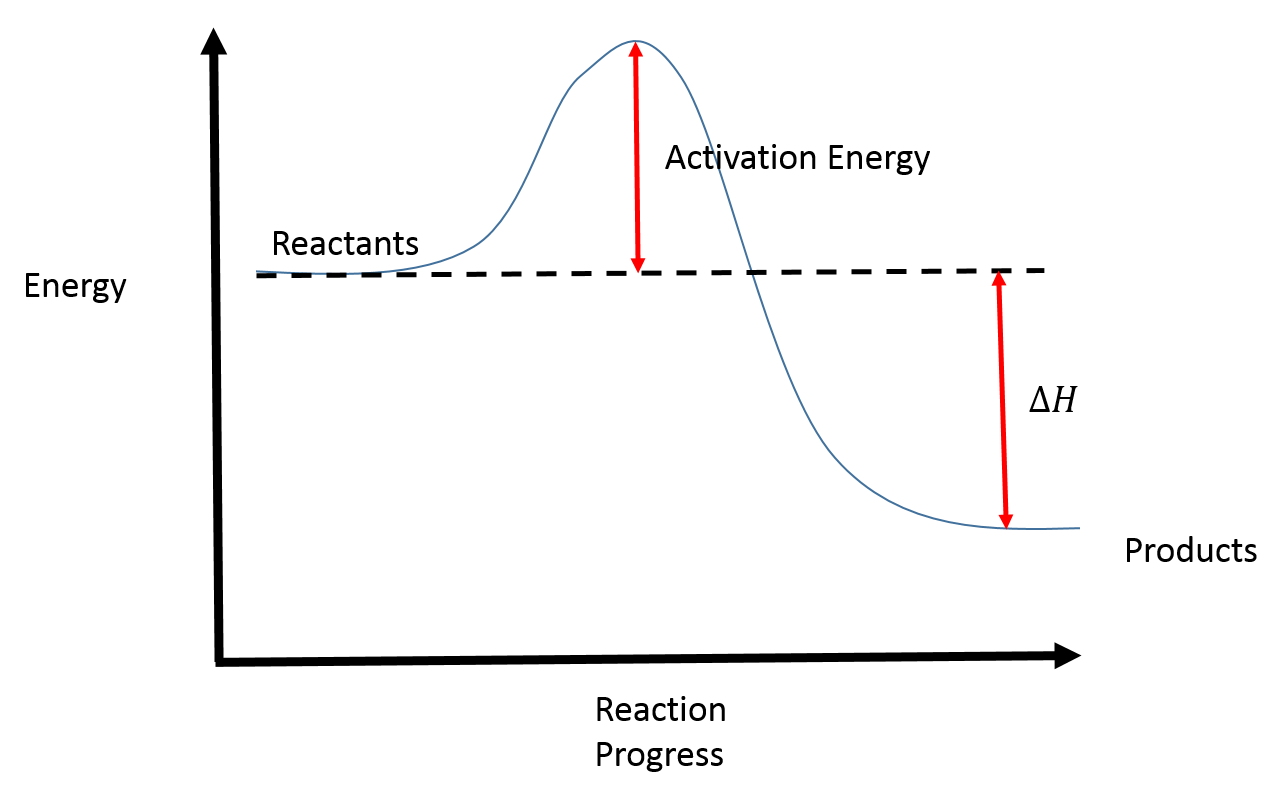The arrow marked in the question represents the activation energy, which is the energy barrier that must be overcome in order for the reactants to form products.

This reaction is also exothermic because the energy of the products is lower than that of the reactants.

### Example Question #12 : College Chemistry

If the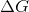of a reversible reaction is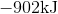, and the activation energy of the forward reaction is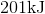, what is the activation energy for the reverse reaction in kilojoules?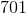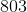The reverse activation energy cannot be determined.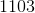Explanation:

Start by drawing and labeling the reaction coordinate diagram.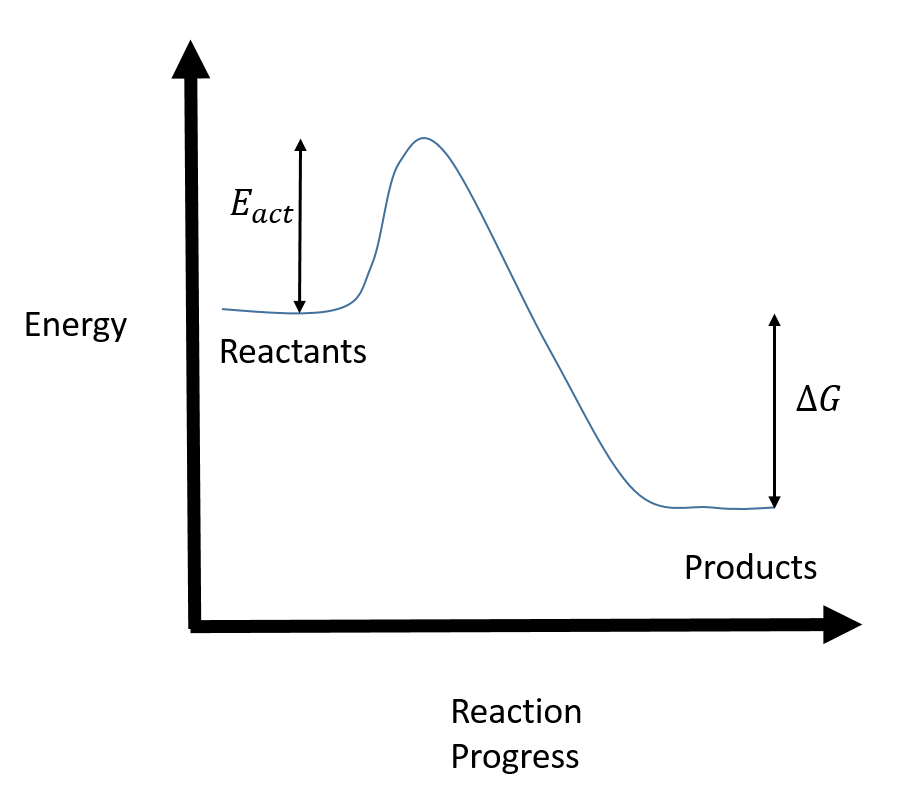Since we know thatis negative, the products must be lower in energy than the reactants.

Now, the question wants you to find the activation energy if we were to reverse the reaction. In other words, we want to start at the products and end up with the reactants. From the diagram, you can see that the reverse activation energy is just the sum of the activation energy for the forward reaction and the.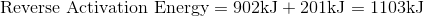### Example Question #13 : College Chemistry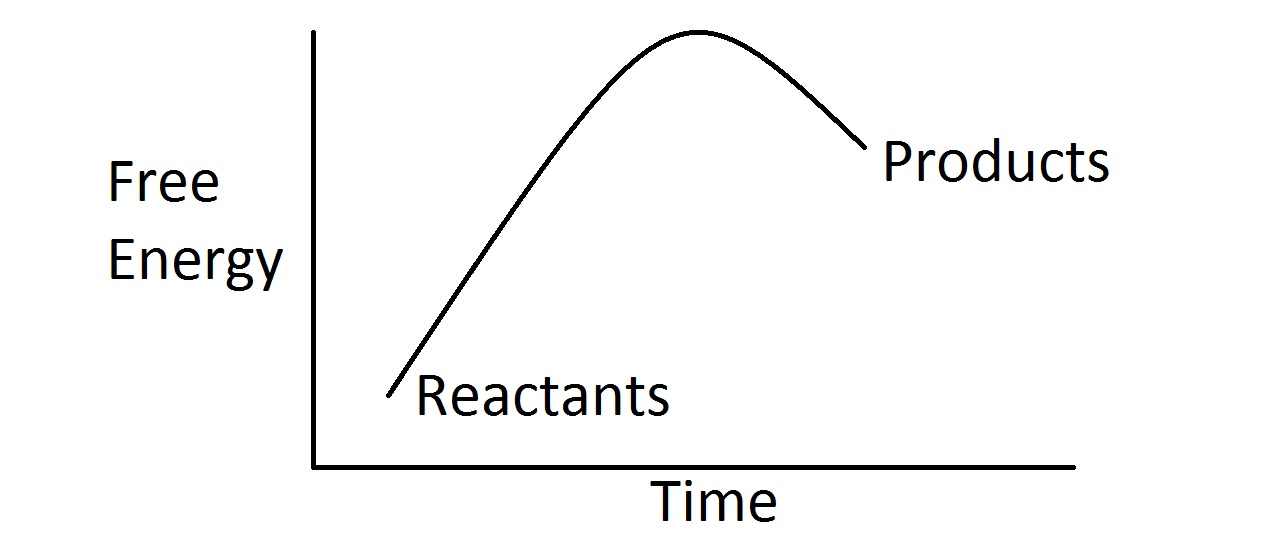Assuming standard conditions, which of the following is a true statement based on the free energy diagram shown?

The entropy of the products is greater than that of the reactants

The entropy of the products is less than that of the reactants

The equilibrium constant for this reaction is less than one

The equilibrium constant for this reaction is greater than one

The equilibrium constant for this reaction is less than one

Explanation:

In this question, we're presented with a free energy diagram for a chemical reaction occurring under standard conditions. We're asked to identify a true statement with regards to this diagram.

Right off the bat, we can see that the free energy of the products is greater than that of the reactants. Thus, this reaction has a positive change in free energy and so it is an endergonic reaction. Recall that one expression for the value of free energy change is as follows.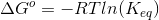From this equation, we can see that the equilibrium constant is related to the standard change in free energy. Moreover, we can see that if the standard free energy change is positive, then the value ofmust be less than. Thus, we know that the reaction is favored towards the reactants.

Additionally, we cannot determine whether the entropy increases or decreases based on the diagram. The reason for this is that entropy is not the only factor that determines the change in free energy of a reaction. The change in enthalpy is also a factor that must be considered.